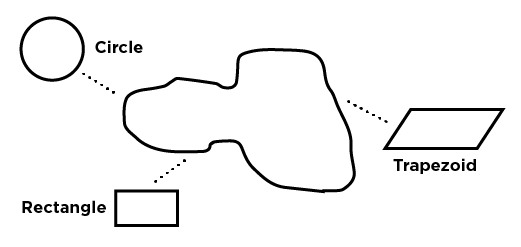## Application Guide

### Calculating the Amount of Nutrient Being Applied

Example: From the above calculations, apply 232 lb per acre of 20-4-4 with 0.86 Team Pro (Rate per acre x percentage nitrogen in 20-4-4) divided by 45.36 = rate nitrogen per 1,000 sq ft (232 x 0.20) / 43.56 = 1.06 lb nitrogen per 1,000 sq ft. (__lbs per acre x __% Nutrient) / 43.56 = __lbs Nutrient applied per 1,000 sq ft OR (Rate per 1,000 x percent. nitrogen in 20-4-4) divided = rate nitrogen per 1.000 sq ft 5.32 x 0.20 = 1.06 lb nitrogen per 1,000 sq ft (__lbs per 1,000 sq ft x __% Nutrient) = __lbs Nutrient per 1,000 sq ft Repeat these calculations for other nutrients in your fertilizer.

### Liquid Fertilizer Nutrients Per Gallon

Find the weight of one gallon of the liquid fertilizer.
Multiply the weight of one gallon by the percentage nutrient to obtain the weight of that nutrient per gallon liquid. Example: 26-3-4 liquid fertilizer weighs 11.0 lbs per gallon 11.0 x 26% nitrogen = 2.81 lbs nitrogen per gallon 26-3-4
11.0 x 3% phosphorus = 0.32 lb phosphorus per gallon 26-3-4
11.0 x 4% potassium = 0.43 lb potassium per gallon 26-3-4
__lbs per gallon x __% Nutrient = __lbs Nutrient per gallon

Determining the rate of fertilizer per 1,000 sq ft based on Nitrogen Needs: Divide the pounds of Nitrogen you need by the percent Nitrogen in the formulation to get pounds of fertilizer you need per thousand square feet.
Example: 25-2-4 fertilizer, need 0.80 lb Nitrogen per thousand 0.8 lbs Nitrogen required / 0.25% Nitrogen in fertilizer = 3.2 pounds 25-2-4 per 1,000 sq ft.
__lbs N / __% N = __lbs per 1,000 fertilizing material to apply Find an open area at least 50 feet long and get a bucket, calculator, tape measure, scale and 25-2-4 fertilizer to be calibrated.
Estimate the amount of 25-2-4 you will need for the test and place this in the spreader.
Set the spreader at the setting suggested for the bag for your spreader. This is a suggested setting only. The condition of your spreader (age and specific variations in your model) will cause differences in the actual setting and this suggested one.
Spread the material along the open area you have chosen. Walk at the pace you normally will during actual applications in yards. Many spreaders are dependent on walking speed along with spreader settings. Start your walk before your measured start point to ensure accurate calculations.
Weigh the amount of material left in the spreader.
Measure the length and width of the area covered. Calculate this into square feet (Length x Width).
Calculate the amount applied per 1,000 sq ft Example: 4.0 lbs applied on 500 sq ft 2.0 lbs x (1,000 sq ft / 500 sq ft) = 4.0 lbs applied per 1,000 sq ft
__lbs x (1,000 sq ft / __sq ft) = __lbs applied per 1,000 sq ft Adjust the spreader setting to adjust your rate to spread less material and repeat the test process to spread 3.2 lbs fertilizer per 1,000 sq ft (to apply 0.8 pounds nitrogen per 1,000 sq ft).
Factors that affect spreader settings include walking speed, differences in granular size, moisture content of granulars, coatings (such as Team Pro), age and condition of spreader, amount of overlap and other similar conditions.

### Calibrating Boom Sprayer Gallons Per Minute

Fill your tank half full with water (to simulate actual conditions). Mark out a strip to be used to measure your speed. Using the throttle and gear settings, measure the amount of time it takes to move through this area, entering the starting point at your regular speed. Calculate your speed in miles per hour. Example: (3,600 x Distance) divided by (5,280 x seconds) = miles per hour
Distance = 200 ft, Time = 34 seconds (3,600 x 200) / (5,280 x 34) = 4.0 miles per hour
(3,600 x __ft) / (5,280 x __seconds) = __miles per hour Make sure all tips on the boom are the same size and rating. Record your setup information: Application volume: gallons per acre or gallons per 1,000 sq ft. ie. 21 gallons per acre
Spray speed: miles per hour...................4 mph
Nozzle spacing: inches...........................20 inches Calculate the required nozzle output. To determine this in gallons per minute (gpm) use one of these formulas: gpm = (gallons per acre x mph x nozzle spacing) divided by 5,980
OR gpm = (gallons per 1,000 sq ft x mph x nozzle spacing) divided by 136 Example: (21 x 4 x 20) / 5,980 = 0.28 gallons per minute
(__gallons per acre x __mph x __inches nozzle spacing) / 5,980 = __gpm
OR (0.48 x 4 x 20) / 136 = 0.28 gallons per minute = 0.28 gpm
(__gallons per 1,000 sq ft x __mph x __inches nozzle spacing) / 136 = __gpm Set the correct pressure. Turn on the sprayer and check for leaks. Inspect and clean tips and strainers. Make sure you have the correct tip for your pressure and application needs as determined by Step 3. Turn on the sprayer and adjust it to the desired pressure, making sure your gauge is accurate.
Check for target flow. Collect water from one new nozzle for 1 minute. Compare this volume with the target flow rate calculated in Step 3. Recheck any rates over or under by 10% of the recommended rate. Fine tune your rate with pressure adjustments. If two or more nozzles are found to be worn, replace all nozzles to ensure consistent, accurate spraying.

### Calibrating Backpack Sprayer Gal Per 1,000 sq ft

Spray an area measuring exactly 1,000 square feet. Walk at a constant speed as you would during normal operations, holding your nozzle at your normal height.
Measure the amount of water left in the sprayer. Subtract this from what you started with. The amount used is the amount sprayed per thousand square feet.
Increase rates by walking more slowly or using a larger tip. Decrease rates by walking faster or using a smaller tip.

For more uniform spray coverage, cover the area twice at half rate, the second pass being at right angle to your first. Example: 3 gallons starting 1 gallon ending
3 - 1 = 2 gallons per 1,000 sq ft being applied
__gallons start - __gallons end = __gallons per 1,000 sq ft (if use 1,000 sq ft as your area) Or if covered an area that was not 1,000 sq ft:
(__gallons start - __gallons end) x 1,000 / __sq ft covered = __gallons per 1,000 sq ft.

### Calibrating Spray Gun Gallons Per Minute

Collect the spray from your gun during one minute of spraying at your normal pressure and tip.
Measure the amount of time needed to walk through 1,000 square feet.
Time needed to cover 1,000 square feet times the amount collected during spraying one minute equals the application rate per 1,000 square feet.
Adjust application rates by changing pressure, application speed or tip size. Example: Collect 1.0 gallons in 1 minute, taking 2.5 minutes to walk 1,000 sq ft 1.0 x 2.5 = 2.5 gallons per 1,000 sq ft being applied (if area covered 1,000 sq ft) __gallons per minute x __minutes walking = __gallons per 1,000 sq ft Or if covered an area that was not 1,000 sq ft:
__gallons per minute x 1,000 / __sq ft covered = __gallons per 1,000 sq ft applied.

## Calculating the Size of An Area

### Square or Rectangle

Length x Width = Area
Example: 40 ft x 30 ft = 1200 sq ft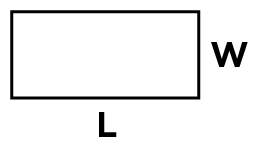### Circle

(EXACT)
pi x r x r = Area pi = 3.1412 r = radius
Example: 3.1412 x 3 ft x 3 ft = 28.27 sq ft
3.1412 x __ft radius x __ft radius = __sq ft Area (within 5% accuracy)
0.8 x D x D = Area
D = Diameter Example: 0.8 x 6 ft x 6 ft = 28.8 sq ft
0.8 x __ft Diameter x __ft Diameter = __sq ft Area### Ovals Or Egg Shapes

(within 5% accuracy)
0.8 L x W = Area
L = Length
W = Width
Example: 0.8 x 30 ft x 70 ft = 1680 sq ft
0.8 x __ft L x __ft W = __sq ft Area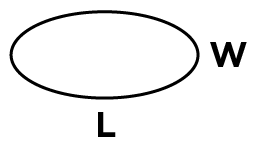### Triangle

0.5 x B x H = Area
B = Base of Triangle
H = Height of Triangle
Example: 0.5 x 75 ft x 245 ft = 9185 sq ft
0.5 x __ft B x __ft H = __sq ft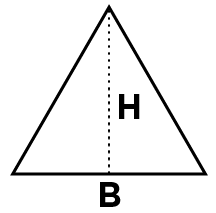### Trapezoid

0.5 (A + B) x H = Area
A = One Parallel Side
B = Second Parallel Side
H = Height Perpendicular to Parallel Sides
Example: 0.5 x (200 ft + 300 ft) x 80 ft = 20,000 sq ft
0.5 (__ft A + __ft B) x __ft H = __sq ft Area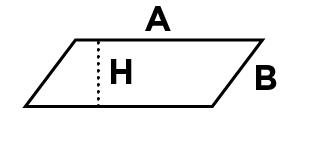### Unusual Shapes

Divide area into sections of shapes you can calculate then add these for total area. Dividing the irregular shape into smaller divisions will result in more accurate calculations than using large areas. Another method is to average lengths and widths to make a calculable shape, being as accurate as possible with these averages to lessen error.